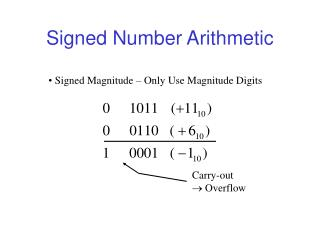Download PresentationSigned Number Arithmetic

Loading in 2 Seconds...

# Signed Number Arithmetic - PowerPoint PPT Presentation

Signed Number Arithmetic. Signed Magnitude – Only Use Magnitude Digits. Carry-out  Overflow. Radix Complement Arithmetic. Radix Complement; In this case 2’s Complement. Carry-out Does NOT Mean Overflow. 2’s Complement Overflow.I am the owner, or an agent authorized to act on behalf of the owner, of the copyrighted work described.
Download Presentation## Signed Number Arithmetic

An Image/Link below is provided (as is) to download presentation

Download Policy: Content on the Website is provided to you AS IS for your information and personal use and may not be sold / licensed / shared on other websites without getting consent from its author.While downloading, if for some reason you are not able to download a presentation, the publisher may have deleted the file from their server.

- - - - - - - - - - - - - - - - - - - - - - - - - - E N D - - - - - - - - - - - - - - - - - - - - - - - - - -
Presentation Transcript
1. Signed Number Arithmetic • Signed Magnitude – Only Use Magnitude Digits Carry-out  Overflow

2. Radix Complement Arithmetic • Radix Complement; In this case 2’s Complement Carry-out Does NOT Mean Overflow

3. 2’s Complement Overflow • If X, Y have opposite signs overflow never occurs whether carry-out exists or not No Carry-out Carry-out • If X, Y have same sign and result sign differs, overflow occurs No Carry-out,Overflow Carry-out, Overflow

4. 1’s Complement Overflow • One’s complement – carry-out indicates a correction is needed • If X>Y, then answer should be X-Y however; registercontains X-Y-ulp since 2n is carry-out bit, therefore must“correct” by adding 1 ulp

5. Example of 1’s Complement Overflow NeedCorrectionSinceOverflow So-called “end-around” carry

6. 1’s Complement Error • One’s Complement Can Be Better Since Easier to Form Complement • Carry-out in One’s Complement Indicates a Correction is Needed Definition • if X>Y, then answer should be X-Y however register contains X-Y-ulp since 2n is carry-out bit, therefore mustcorrect by adding 1 ulp to answer

7. “End-Around” Carry Design Carry-out • This is “end-around” carry – always add carry-out to LSD

8. Other Number Systems • Binary Number Systems are Most Common • In terms of building “fast” systems, we should consider: • Negative Radix • Signed Digit • Log (logarithm) • Signed Log • Complex Radix • Mixed Radix • Residue Number Systems

9. Negative Radix Fixed Position Systems Nega-decimal example:

10. Nega-Decimal Number System Largest Positive Value, Xmax: Smallest Value, Xmin: Finite Register Length, n=3 digits: Asymmetric System!!!: 10 times more positive than negative values represented

11. Nega-Decimal Number System Finite Register Length, n=4 digits: Now more Negative Values than Positive Nega-decimal System Characteristics: • Arithmetic Operations Same Regardless of Sign of Number • No Signed Digit/Complement Representation Needed • Sign of X Determined by Position of First Non-zero Digit

12. Nega-Binary Number System Negative Radix: Example How is this Addition Operation Performed?????

13. Nega-Binary Number System wi Values (5)10 (-3)10 (1+1=4-2)10 (0+0=0)10 (4+4=16-8)10 (0-8=-8)10 (5-3=2)-10 Carry-out

14. Nega-Binary Adder Design • Individual Adder Cells Produce Two Carry-out Bits • Design a Circuit at Gate Level for a 4-Digit Nega-Binary Adder • Hint: Cout Functions Should Look Familiar!